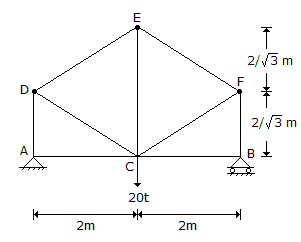# Civil Engineering - UPSC Civil Service Exam Questions

11.

A pin-jointed truss is loaded as shown in the given figure. The force in member CE isA. 10 tonnes tensile B. 10 tonnes compressive C. 5 tonnes tensile D. 5 tonnes compressive

Explanation:

No answer description available for this question. Let us discuss.

12.

What type of noise can be abated by providing lining on walls and ceiling with sound absorbing material?

 A. Source noise B. Reflection noise C. Structural noise D. Direct air-borne noise

Explanation:

No answer description available for this question. Let us discuss.

13.

Which of the following treatment(s) will be indicated for a rural water supply from a deep groundwater source ?
1. Sedimentation.
2. Alum dosage.
3. Potassium permanganate dosing.
4. Bleaching powder application.
Select the correct answer using the codes given below :

 A. 1, 2 and 3 B. 1, 2 and 4 C. 3 and 4 D. 4 alone

Explanation:

No answer description available for this question. Let us discuss.

14.

Maximum gross take-off weight of an aircraft is

 A. equal to the maximum structural landing weight B. less than the maximum structural landing weight C. more than the maximum structural land weight D. equal to the empty operating weight plus the payload

Explanation:

No answer description available for this question. Let us discuss.

15.

For a circular column having its ends hinged, the slenderness ratio is 160. The l/d ratio of the column is

 A. 80 B. 57 C. 40 D. 20